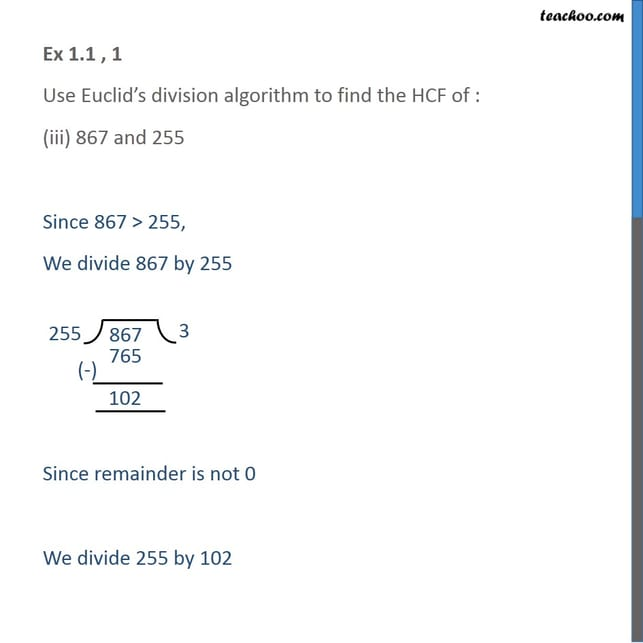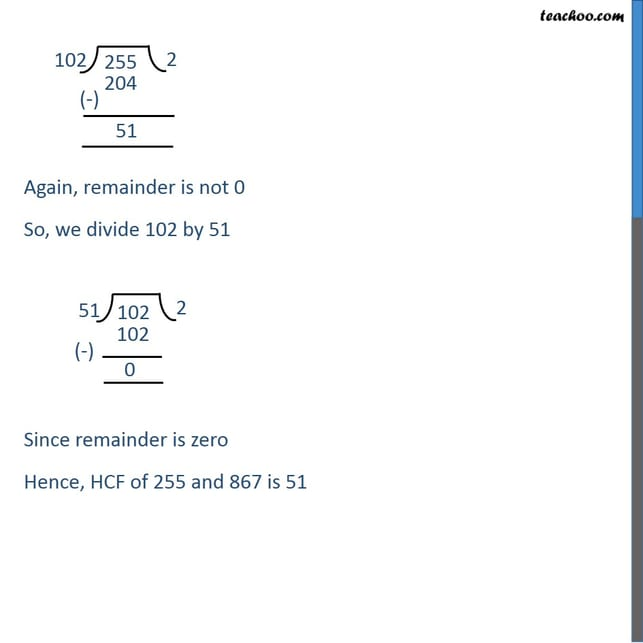Euclid's Division Algorithm

Chapter 1 Class 10 Real Numbers
Serial order wiseLearn in your speed, with individual attention - Teachoo Maths 1-on-1 Class

### Transcript

Ex 1.1 , 1 Use Euclid’s division algorithm to find the HCF of : (iii) 867 and 255 Since 867 > 255, We divide 867 by 255 Since remainder is not 0 We divide 255 by 102 Again, remainder is not 0 So, we divide 102 by 51 Since remainder is zero Hence, HCF of 255 and 867 is 51